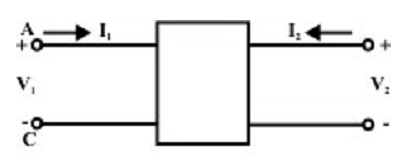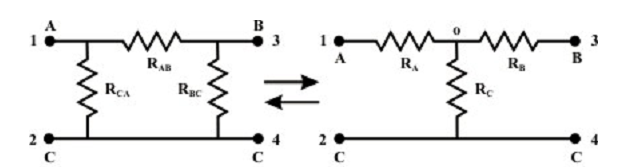## Wye -Delta OR Delta -Wye Transformations Assignment Help

Assignment Help: >> Electrical Engineering - Wye -Delta OR Delta -Wye Transformations

Wye (Y) - Delta (Δ) OR Delta (Δ)-Wye (Y) Transformations

There are some circuit configurations which cannot be simplified by series-parallel combination only. A simple transformation depend on mathematical method is readily simplifies the electrical circuit configuration. The circuit configuration is as shown belowFigure: One port network

is a common one-port circuit. Whenever any voltage source is associated across the terminals, the current entering via any one of the two terminals, equivalents the current leaving the other terminal. For illustration, inductance, resistance, and capacitance act as a one-port. On the other hand, the two-port is a circuit containing two pairs of terminals. Each pair acts as a one-port; current entering in one terminal should be equivalent to the current living the other terminal.Figure: Two port network

The figure shown above can be explained as a four terminal network, for ease subscript 1 to refer to the variables at the input port (i.e., at the left) and the subscript 2 to refer to the variables at the output port (i.e., at the right). The most significant subclass of two-port networks is the one in which the minus reference terminals of the input and output ports are at similar. This circuit configuration is readily possible to consider the ‘or π Δ’ – network also as a three-terminal network in the figure below. Another often encountered circuit configuration which shown in figure below is around termed to as a three-terminal Y connected circuit and also two-port circuit.The name derives from the configuration or shape of the circuit diagrams, that look correspondingly like the letter Y and the Greek capital letter Δ.

 Delta – Wye conversion Transform from Delta to Star or Wye Transform from Star or Wye to Delta